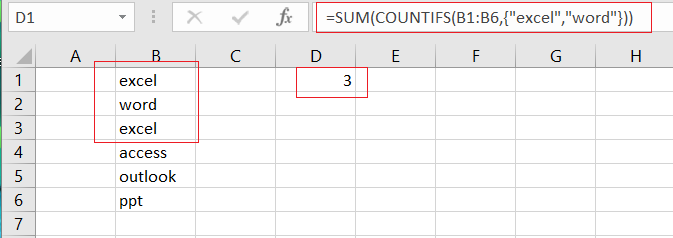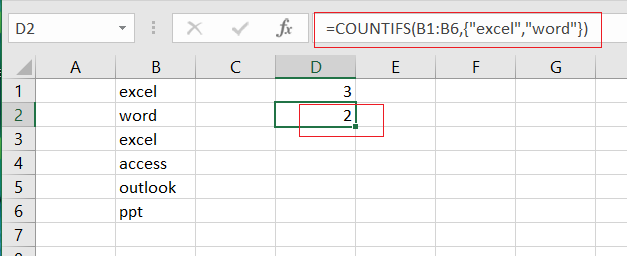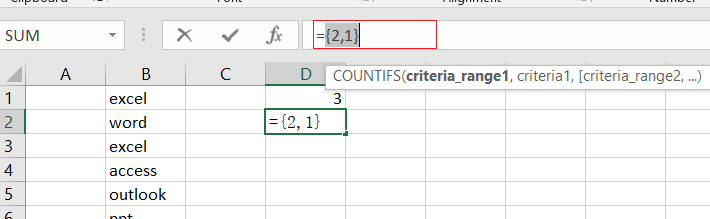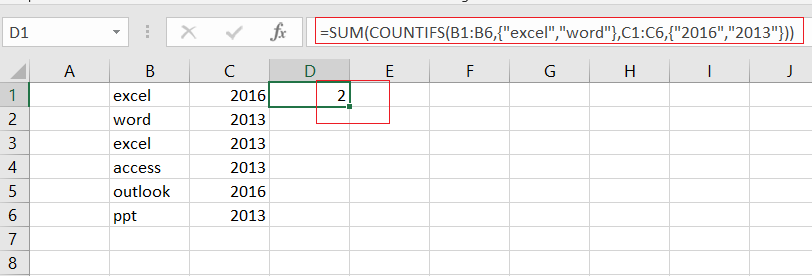# How to Use COUNTIFS function with Multiple Criteria and OR Logic in Excel

This post will guide you how to Use COUNTIFS function with multiple criteria and OR logic in Excel 2013/2016 or Excel office 365. How to count with multiple criteria and OR logic in Excel.

## COUNTIFS with Multiple Criteria and OR Logic

Normally, when you provide multiple conditions to a COUNTIFS function, and it will check all the conditions and returns rows which matches all the conditions, it means that it runs on AND logic.

If you want to count with multiple conditions and OR logic in your formula, and you can also use the COUNTIFS function to achieve OR logic. Then you still need to use the SUM function and array with multiple condition.

The generic formula is as below:

=SUM(COUNTIFS(range1,{criteria1,criteria2},range2,{criteria3,criteria4}…))

## Example

Assuming that you have a list of data in range B1:B6 in your worksheet, and you wish to count product name which is “excel” or “word”, and you can use the COUNTIFS function with an array constant. The formula is like this:

=SUM(COUNTIFS(B1:B6,{“excel”,”word”}))Let’s See That How This Formula Works:

=COUNTIFS(B1:B6,{“excel”,”word”})The COUNTIFS function can be used to match each item of condition array {“excel”, “word”} and return count of each item in another array list. It means that it will return two values, one is a count for “excel” and another is a count for “word”, the array result is like this:

{2,1}Finally, you can use the SUM function to add up all items in an array.

## Adding Two or Multiple Criteria with OR Logic

You can also add two or more criteria to this formula to count multiple criteria using COUNTIFS function with OR logic in Excel. You ‘ll need to add another single column array for one or add another single column array for the other criteria. For example, you wish to count product names that are “excel” or “word”, another criteria is “2016” or “2013”, you can use the below formula:

=SUM(COUNTIFS(B1:B6,{“excel”,”word”},C1:C6,{“2016″,”2013”}))### Related Functions

• Excel COUNTIFS function
The Excel COUNTIFS function returns the count of cells in a range that meet one or more criteria. The syntax of the COUNTIFS function is as below:= COUNTIFS(criteria_range1, criteria1, [criteria_range2, criteria2]…)…
• Excel SUM function
The Excel SUM function will adds all numbers in a range of cells and returns the sum of these values. You can add individual values, cell references or ranges in excel.The syntax of the SUM function is as below:= SUM(number1,[number2],…)